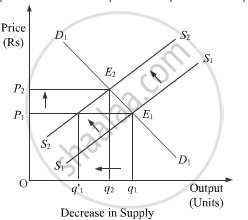# The Market for a Good is in Equilibrium. How Would an Increase in an Input Price Affect the Equilibrium Price and Equilibrium Quantity, Keeping Other Factors Constant? Explain Using a Diagram. - Economics

The market for a good is in equilibrium. How would an increase in an input price affect the equilibrium price and equilibrium quantity, keeping other factors constant? Explain using a diagram.

#### Solution

An increase in input price results in decreases in the supply and no change in demand. The given figure shows how would an increase in an input price affect the equilibrium price and equilibrium quantity:The market supply falls with the rise in the input prices. As a result, the supply curve shifts parallelly leftwards to S2S2 from S1S1. Thus, at the initial price OP1, holding demand unchanged, there exist excess demand equivalent to (Oq– Oq1') units of output. This excess demand will lead some of the consumers to pay a higher price in order to obtain the extra units of output. The rise in the market price will continue until it reaches OP2, where the new supply curve S2S2 intersects the initial market demand curve D1D1. The new equilibrium is established at point Ewith the equilibrium price as OP2and equilibrium output as Oq2. At the new equilibrium, the equilibrium price has risen, whereas, the equilibrium quantity has fallen.
To summarise,
Decrease in supply(due to a rise in the input prices) ⇒ Excess demand at the existing price ⇒ Rise in the price level ⇒ New equilibrium ⇒ Rise in price and fall in quantity demanded.

Concept: Equilibrium Price
Is there an error in this question or solution?